Loading World Series Futures...DraftKings World Series Odds
+350
3.5 to 1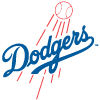Dodgers
16.7% implied probability

+550
5.5 to 1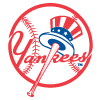Yankees
11.6% implied probability

+800
8 to 1Padres
8.3% implied probability

+1000
10 to 1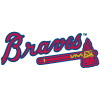Braves
6.8% implied probability

+1000
10 to 1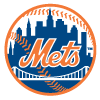Mets
6.8% implied probability

+1100
11 to 1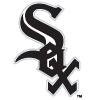White Sox
6.3% implied probability

+1400
14 to 1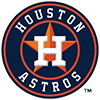Astros
5.0% implied probability

+1800
18 to 1Twins
4.0% implied probability

+2200
22 to 1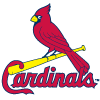Cardinals
3.3% implied probability

+2200
22 to 1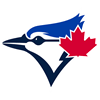Blue Jays
3.3% implied probability

+2500
25 to 1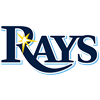Rays
2.9% implied probability

+2800
28 to 1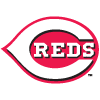Reds
2.6% implied probability

+2800
28 to 1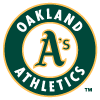Athletics
2.6% implied probability

+3300
33 to 1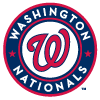Nationals
2.2% implied probability

+3800
38 to 1Angels
1.9% implied probability

+4000
40 to 1Indians
1.8% implied probability

+4000
40 to 1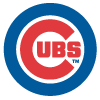Cubs
1.8% implied probability

+4000
40 to 1Phillies
1.8% implied probability

+4400
44 to 1Brewers
1.7% implied probability

+5000
50 to 1Red Sox
1.5% implied probability

+7500
75 to 1Marlins
1.0% implied probability

+8000
80 to 1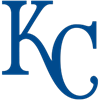Royals
0.9% implied probability

+8000
80 to 1Giants
0.9% implied probability

+10000
100 to 1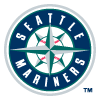Mariners
0.7% implied probability

+10000
100 to 1Rockies
0.7% implied probability

+10000
100 to 1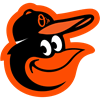Orioles
0.7% implied probability

+10000
100 to 1Diamondbacks
0.7% implied probability

+15000
150 to 1Tigers
0.5% implied probability

+15000
150 to 1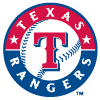Rangers
0.5% implied probability

+25000
250 to 1Pirates
0.3% implied probability